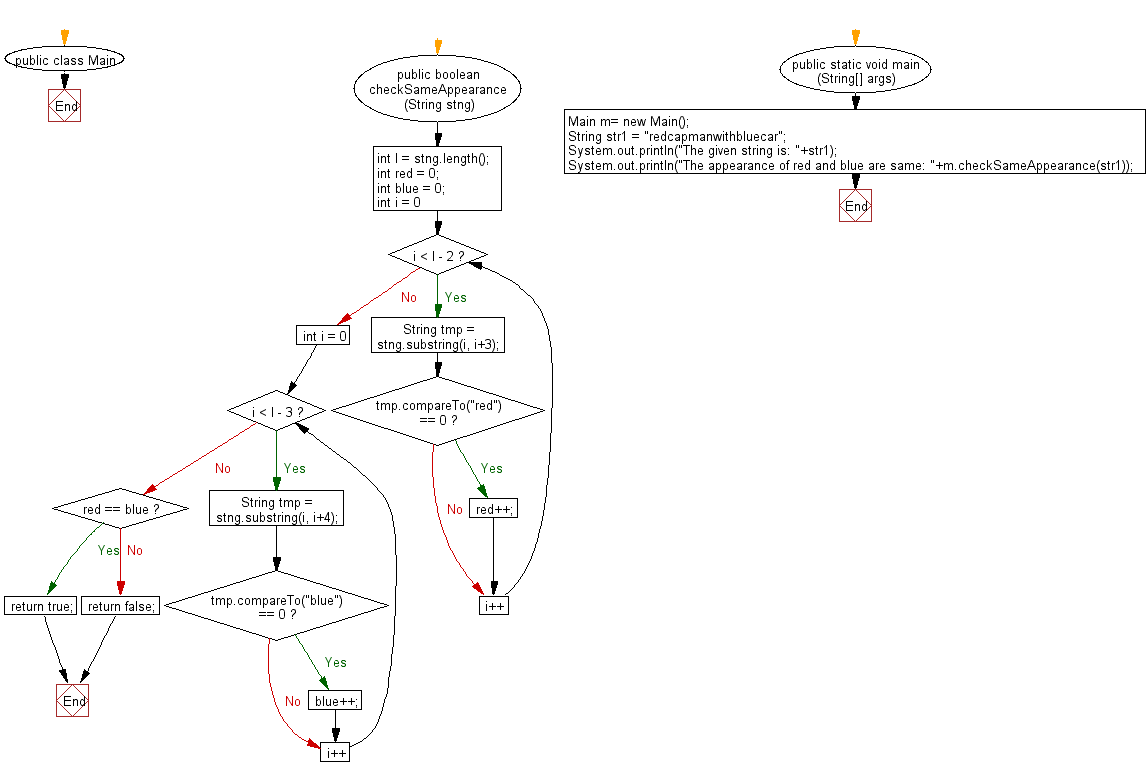﻿ Java exercises: Check whether the string 'red' and 'blue' appear in same number of times in a given string - w3resource# Java String Exercises: Check whether the string 'red' and 'blue' appear in same number of times in a given string

## Java String: Exercise-81 with Solution

Write a Java program to check whether the string 'red' and 'blue' appear in same number of times in a given string.

Sample Solution:

Java Code:

``````import java.util.*;
public class Main
{
public boolean checkSameAppearance(String stng)
{
int l = stng.length();
int red = 0;
int blue = 0;
for (int i = 0; i < l - 2; i++)
{
String tmp = stng.substring(i, i+3);
if (tmp.compareTo("red") == 0)
red++;
}
for (int i = 0; i < l - 3; i++)
{
String tmp = stng.substring(i, i+4);
if (tmp.compareTo("blue") == 0)
blue++;
}

if (red == blue)
return true;
else
return false;
}

public static void main (String[] args)
{
Main m= new Main();
String str1 =  "redcapmanwithbluecar";
System.out.println("The given string is: "+str1);
System.out.println("The appearance of red and blue are same: "+m.checkSameAppearance(str1));
}
}

``````

Sample Output:

```The given string is: redcapmanwithbluecar
The appearance of red and blue are same: true
```

Pictorial Presentation:Flowchart:Java Code Editor:

Improve this sample solution and post your code through Disqus

What is the difficulty level of this exercise?

﻿